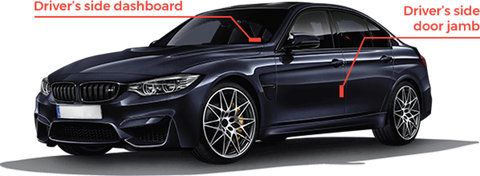# How to Find the VIN (Chassis Number) on Your BMW

###### Mar 2, '21

North American BMW vehicles (common locations):

1. The driver side windshield, bottom right facing outward.

2. Inside the driver's side door jamb.

European BMW vehicles (common locations):

1. Stamped onto the right-hand suspension turret in the engine bay.

2. A VIN code 'plate' is riveted to the left-hand suspension turret in the engine bay. This plate may shows additional information such as weight and capacity.

3. The passenger side windshield, bottom right facing outward.## How to Read BMW VIN Codes

The following is the standardized structure of the VIN for the United States and Canada.

Char 1: Country of Manufacturer
1 = USA 2 = Canada 3 = Mexico 4 = USA J = Japan K = Korea S = England W = Germany Z = Italy
Char 2: Vehicle Manufacturer Code
A = Audi B = BMW H = Honda A = Jaguar D = Mercedes N = Nissan T = Toyota V = Volvo V = VW
Char 3: Vehicle Category or Manufacturing Division
Vehicle category type or manufacturing division, or can be both.
Char 4-8: Features & Attributes
Codes for body style, engine type, model, series, etc. Varies among models and factory installed equipment.
Char 9: Digit Calculation Check
VIN number accuracy check digit, compulsory for vehicles in North America, but often used world-wide.
Char 10: Model Year Encoding
A = 1980 B = 1981 C = 1982 D = 1983 E = 1984 F = 1985 G = 1986 H = 1987 J = 1988
K = 1989 L = 1990 M = 1991 N = 1992 P = 1993 R = 1994 S = 1995 T = 1996 V = 1997
W = 1998 X = 1999 Y = 2000 1 = 2001 2 = 2002 3 = 2003 4 = 2004 5 = 2005 6 = 2006
7 = 2007 8 = 2008 9 = 2009 A = 2010 B = 2011 C = 2012 D = 2013 E = 2014 F = 2015
G = 2016 H = 2017 J = 2018 K = 2019 L = 2020 M = 2021 N = 2022 P = 2023 R = 2024
Char 11: Vehicle Identifier Section (1)
Number reveals the assembly plant for the vehicle.
Char 12-17: Vehicle Identifier Section (2)
Used by the manufacturer to identify an individual vehicle. May include information on options installed, engine and transmission choices, but often is a simple sequential number.